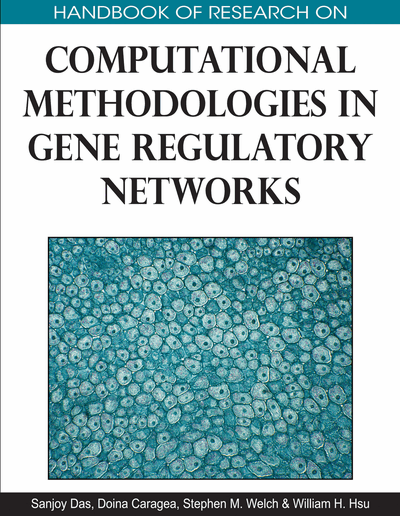# Bayesian Networks for Modeling and Inferring Gene Regulatory Networks

Sebastian Bauer (Charité Universitätsmedizin Berlin, Germany) and Peter Robinson (Charité Universitätsmedizin Berlin, Germany)
DOI: 10.4018/978-1-60566-685-3.ch003
Available
\$37.50
No Current Special Offers

## Abstract

Bayesian networks have become a commonly used tool for inferring structure of gene regulatory networks from gene expression data. In this framework, genes are mapped to nodes of a graph, and Bayesian techniques are used to determine a set of edges that best explain the data, that is, to infer the underlying structure of the network. This chapter begins with an explanation of the mathematical framework of Bayesian networks in the context of reverse engineering of genetic networks. The second part of this review discusses a number of variations upon the basic methodology, including analysis of discrete vs. continuous data or static vs. dynamic Bayesian networks, different methods of exploring the potentially huge search space of network structures, and the use of priors to improve the prediction performance. This review concludes with a discussion of methods for evaluating the performance of network structure inference algorithms.
Chapter Preview
Top

## Background

### Biology

GRNs coordinate the changes in cellular behavior associated with development or response of the cell or organism to extracellular stimuli. Transcription factors are the molecules that activate or repress downstream genes by binding to promoter and other sequences (cis-regulatory modules) of genes, thereby modulating the rate of transcription of genes. Combinations of transcription factor binding events in any one promoter are one of the important factors determining the level of the corresponding mRNA in the cell. The regulatory state of the cell has been described as the total set of active transcription factors. However, a number of other molecules influence the activation state and concentration of transcription factors. For instance, signaling pathways consisting of ten or hundreds of proteins can transduce an extracellular event (such as the binding of a ligand to a receptor) into an intracellular biochemical signal by cascading protein modification events. For instance, a receptor-ligand binding event may induce phosphorylation (and activation) of an intracellular signaling molecule, which in turn phosphorylates other molecules, thereby propagating the signal through a cascade or network of proteins, some of which activate transcription factors and thereby influence the transcription of target genes. Other factors, such as non-coding RNAs, histone modifications, and CpG methylation, can also influence the level of mRNA of target genes. Therefore, measurement of mRNA levels can provide only a partial view of the regulatory state of a cell. At present, however, there remain major technical difficulties in obtaining large-scale measurements of protein levels or protein modifications, so that network structural inference has for the most part been attempted with mRNA data.

### Graph Theory

Graphs are abstract entities of discrete mathematics which are used to encode relationships of interest between objects of the same domain. Formally, a graph is a pair G=(V,E), in which V is finite set of vertices, representing the objects, and E a set of pairs of distinct elements of V, which is a binary relation over V. Elements of E are called edges (or arcs). The pairs may be ordered or not. An order implies a direction. If all edges of G are directed, the graph is directed. If at least one edge is directed we call the graph a partially directed graph. Otherwise the graph is an undirected graph.

A path with length n is a sequence of vertices (v1,...,vn) which respects the edges, i.e.,for all i. A cycle is a special path whose start vertex v1 equals to the end vertex vn. A directed path is a path, in which the edges between the vertices are all directed. A directed graph is acyclic if it contains no directed cycle; such graphs are referred to as directed acyclic graphs (DAGs). A partially directed acyclic graph (PDAG) is a graph which contains directed and undirected edges, but which doesn't contain any directed cycle.

## Key Terms in this Chapter

Sparse Candidate Algorithm: The SCA is an approximation algorithm for the problem of finding a structure of a Bayesian network that maximizes a given Bayesian scoring metrics. It employs the feature that biological networks are usually sparse and consists of two phases, the restriction and the maximization phase.

Priors: A prior can be specified during a learning procedure that takes advantage of Bayes’ theorem and may represent properties that are already known and therefore don’t need to be rediscovered. It is especially useful when data is sparse, which is the case in micro array analysis, as it can significantly reduce the space of all DAGs that is used during the search.

Bayesian Scoring Metrics: A Bayesian Scoring Metric is a function that scores how well a given graph explains given data.

MCMC: The MCMC (Markov chain Monte Carlo) is a procedure which allows sampling instances from complex probability distribution. With respect to GRNs MCMC is used to sample from the space of all DAGs whereby the sampling scheme follows a distribution that is based on a Bayesian scoring metrics. Thus more probable DAGs, that is, DAGs that may better explain the data, are sampled more often and therefore one can construct a likely network structure.

Bayesian Networks: A Bayesian network is a probabilistic graphical model. It contains of a graph whose vertices represent variables, for instance random variables. The directed edges of the graph encode direct dependency relation of one variable to another. Bayesian networks can be used to predict the state of variables, when other variables are fixed. In addition, Bayesian networks can be learned from sampled data.

## Complete Chapter List

Search this Book:
Reset